# Singularities of Cubic Surface

Cubic surfaces are implicit surfaces in projective three space with terms which are degree three or less. These surfaces can have several different types of singularity from the basic conical singularity A1 to those containing a cross-caps and a line of nodal points.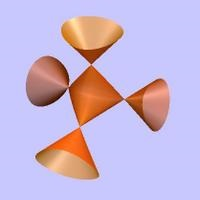## Projective space

Cubic surfaces are defined in projective space which is a lot like normal 3D space, but which some extra structure at infinity. Points in projective 3 space are specified by 4 number, written (x:y:z:w), with an equivalence relation so that all non zero multiples of a point are considered equivalent. Hence, (ax: ay: az: aw)=(x:y:z:w) for all not zero a. In particular if w is not zero then (x:y:z:w)=(x/w:y/w:z/w:1) and dropping the final number projects this to normal R3 so (x:y:z:w) is projected to (x/w,y/w,z/w) allowing the surface to be view in three dimensions.

One way to think of the space is the set of lines through the origin in R4. If (x, y, z, w) is a points on such a line then so is (a x, a y, a z, a w) and the equivalence relation follows. Alternatively the space can be though of as the three sphere S3 with antipodal points identified.

For most of the surfaces below we have used a projection so that the points (1:0:0:0), (0:1:0:0), (0:0:1:0), (0:0:0:1) map to the vertices of a tetrahedron (1,1,1), (-1,-1,1), (1,-1,-1), (-1,1,-1). This is a particularly useful projection as the singularities of the expressions given by Cayley tend to lie at these four points.

## Singularities

The type of singularity is determined by a classification due to Arnol'd, and are specified by their normal forms. In the complex case there is just one normal form for each, but in the real case changing the signs of the terms produce different images. Some of the alternate forms are shown, for many singularities one form is a single point, which is not shown.

TypeNormal formCurve in 2DSurface in 3DCoxeter diagram
Simple singularities
A1 x2+y2+z2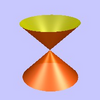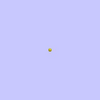A2 x3+y2+z2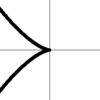A3 x4+y2+z2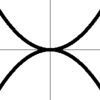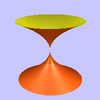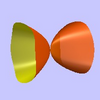A4 x5+y2+z2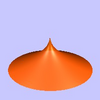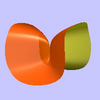A5 x6+y2+z2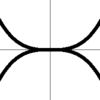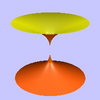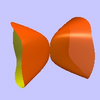D4 x3+x y2+z2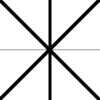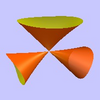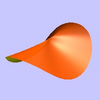D5 x4+x y2+z2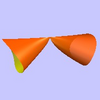E6 x3+y4+z2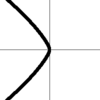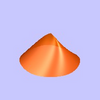Non simple singularities
6 x3+y3+z3
+k x y z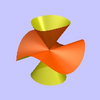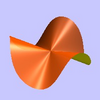## Coxeter–Dynkin diagram and the ADE classification

The various cubic surfaces can be characterised by their Coxeter–Dynkin diagram. These diagrams show the relationship between the generators of the Coxeter groups of symmetries, and are also used to describe the homology groups of cubic surfaces.

The Coxeter–Dynkin diagram of the different cubic surfaces are all derived from the diagram of the ${\tilde {E}}_{6}$ Affine Coxeter Group, by removing some of the edges.For example, and A1 singularity is represented by a single node and an A2 singularities is represented by a two nodes joined by a line. Hence for the Cubic with two A1 singularites and one A2 singularity is represented by the diagramMy page Singularities and the ADE classification tried to explain this characterisation in a visual manner.

## The Cubic Surfaces

The following list of singularities follows Cayley. Schlafli was first to classify the various types. We use the modern notation for singularities following Bruce and Wall. Holzer and Labs distinguish between the different forms of the real singularities giving 45 types with rational double points.

Capitals X, Y, Z, W are used for coordinates in projective space and x, y, z for coordinates in R3.

### Class I: No singularities

There are a number of well known non singular cubics.

Clebsch surface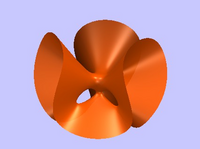This surface contain the maximum possible 27 real lines.

X3+Y3+Z3+W3-(X+Y+Z+W)3=0

One projection is 16 x3 + 16 y3 - 31 z3 + 24 x2 z - 48 x2 y - 48 x y2 +24 y2 z - 54 √3 z2 - 72 z

Fermat Cubic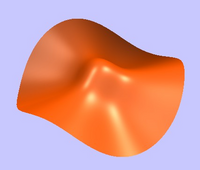X3+Y3+Z3+W3=0

Ref: Wikipedia

### Class II: one A1 singularity

In Cayley's notation: C2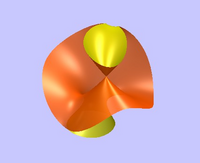W (a X2 + b Y2 + c Z2 + f X Y+ g X Z+h Y Z)+2 k X Y Z### Class III: one A2 singularity

In Cayley's notation: B3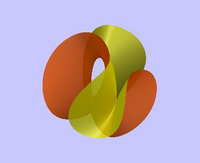2W(X+Y+Z)(l X+m Y+n Z) + 2 k X Y ZSome nice examples includex z + y2(x+y+z)

By Bruce Hunt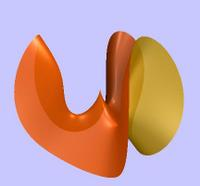S11

x2+y2+z3+3.2 (x3-3 x y2)

### Class IV: two A1 singularities

In Cayley's notation: 2 C2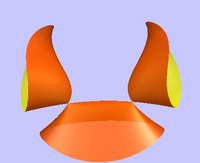W X Z+Y2(k Z+l W)+a X3 +b X2 Y+c X Y2 + d Y3### Class V: one A3 singularity

In Cayley's notation: B4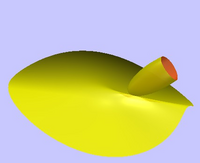W X Z +(X+Z)(Y2-a X2-b Z2)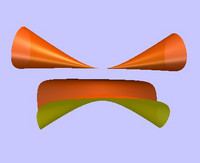(x2 + y2) w + 2 x (z2 - 2 x2 - 4 y2); w=1-y

Number KM26 in Holzer and Labs

### Class VI: one A1 and and one A2 singularities

In Cayley's notation: B3+C2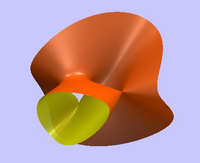W X Z+Y2 Z+a X3 +b X2 Y+c X Y2 + d Y3### Class VII: one A4 singularity

In Cayley's notation: B5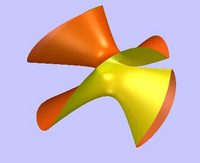W X Z +Y2 Z +Y X2-Z3### Class VIII: three A1 singularities

In Cayley's notation: 3 C2Y3+Y2(X+Z+W)+4 a X Z W### Class IX: two A2 singularities

In Cayley's notation: 2 B3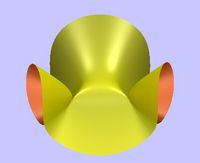W X Z+a X3+ b X2 Y + c X Y2 + d Y3### Class X: one A1 and one A3 singularities

In Cayley's notation: B4+C2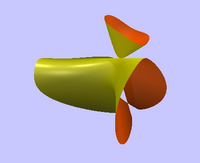W X Z+(X+Z)(Y2-X2)### Class XI: one A5 singularity

In Cayley's notation: B6W X Z + Y2 Z+X3- Z3### Class XII: one D4 singularity

In Cayley's notation: U6W(X+Y+Z)2+X Y ZTwo nice examples of the surface show the different real forms of the singularity.S16

z2 ( z + 4) + y (y - √3) x) ( y + √3 x)S17

z2 ( z + 4) + y (x2 + y2)

### Class XIII: two A1 and one A2 singularities

In Cayley's notation: B3+2 C2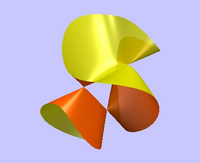W X Z+Y2(X+Y+Z)### Class XIV: one A1 and one A4 singularities

In Cayley's notation: B5+C2W X Z+Y2 Z+Y X2### Class XV: 1 D5 singularity

In Cayley's notation: U7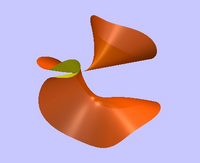W X2+X Z2+Y2 Z### Class XVI: four A1 singularities

In Cayley's notation: 4 C2W(X Y+X Z+Y Z)+X Y ZCayley's Cubic4 (x2+y2+z2) + 16 x y z - 1;

Cayley's expression X Y Z + X Y W + X Y W + Y Z W=0 has nodes at the four points (1:0:0:0), (0:1:0:0), (0:0:1:0) and (0:0:0:1) we can apply a rotation so that these points map to four verticies of cube center at (0:0:0:1), that is the points (1:1:1:1), (-1:-1:1:1), (1:-1:-1:1), (1:-1:-1:1), (-1:1:-1:1). Projecting these points to R3 via (x:y:z:w) → (x/w,y/w,z/w) gives vertices on a cube center origin. Plotting the resulting expression

X Y Z + X Y W + X Z W + Y Z W;
X = x + y + z + w;
Y = -x - y + z + w;
Z = x -y -z + w;
W = -x + y - z + w;
w = 1;

gives a surface where the nodal points are clearly visable.S6

-x2 + z x2 + y2 z - 2 z3 + 3 z2 - z;

A different projection of the same surface. From University of Turin

### Class XVII: one A1 and two A2 singularities

In Cayley's notation: 2 B3 + C2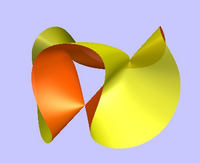W X Z+X Y2+Y3### Class XVIII: two A1 and one A3 singularities

In Cayley's notation: B4 +2 C2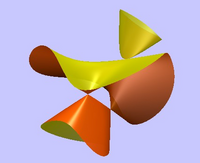W X Z+(X+Z)Y2### Class XIX: one A1 and one A5 singularity

In Cayley's notation: B6 + C2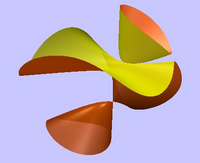W X Z + Y2 Z + X3### Class XX: one E6 singularity

In Cayley's notation: U8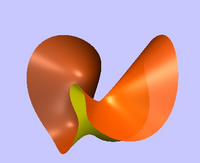W X2 + X Z2 + Y3### Class XXI: three A2 singularities

In Cayley's notation: 3 B3W X Z+Y3Cayley 1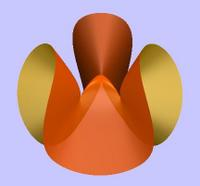p1 + (a2z+a3)p3 + a4 z3 + a5z2 + a6 z + a7;
p1=2 x3-6 x y2;
p3=x2+y2;
a1 = -1; a2 = -1; a3=-3 a1;
a4 = 1; a5=a22/(3 a1);
a6=-a2; a7=a1;

### Class XXII: two cross-caps and a nodal line

In Cayley's notation: S(1,1)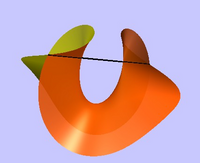W X2 + Z Y2

### Class XXIII: nodal line

In Cayley's notation: S(1,1)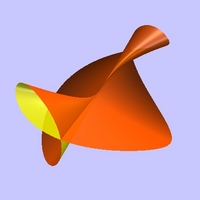X(W X+Y Z)+Y3

## References

• Bruce, J. W.; Wall, C. T. C. (1979), "On the classification of cubic surfaces", The Journal of the London Mathematical Society. Second Series 19 (2): 245–256, doi:10.1112/jlms/s2-19.2.245, MR533323, ISSN 0024-6107 - Has details on the modern classification.
• Cayley, Arthur (1869), "A Memoir on Cubic Surfaces", Philosophical Transactions of the Royal Society of London (The Royal Society) 159: 231–326, ISSN 0080-4614 - The classification this page is based on.
• Holzer, Stephan; Labs, Oliver, "Illustrating the Classification of Real Cubic Surfaces" PDF - Visulise the 45 topological types for real cubic surfaces.
• La collezione dei modelli di superfici del Dipartimento di Matematica dell'Università di Torino. (The Models of the Department of Mathematics University of Turin). Original page is dead, a mirror can be found at Gian Marco Todesco personal page. - A collection of 30 models originally done in plaster, cover many of the surfaces seen here.
• H. Knörrer and T. Miller. Topologische Typen reeller kubischer Flächen. Mathematische Zeitschrift, 195, 1987. - Classification of the 45 real types.
• Cubic surface home page - A website dedicated to cubic surfaces. Has images of each type.

## 3D Viewer-Generator

Controls Java Version

Click image on left to load 3D model. Rotate with mouse, hold 's' and drag with mouse to scale, hold 't' and drag with mouse to translate.

3D surfaces are generated by the SingSurf algebraic surface program The raytraced images have been produced using Surf, using SingSurf and Javaview to select good views for raytracing.

Msqli defined